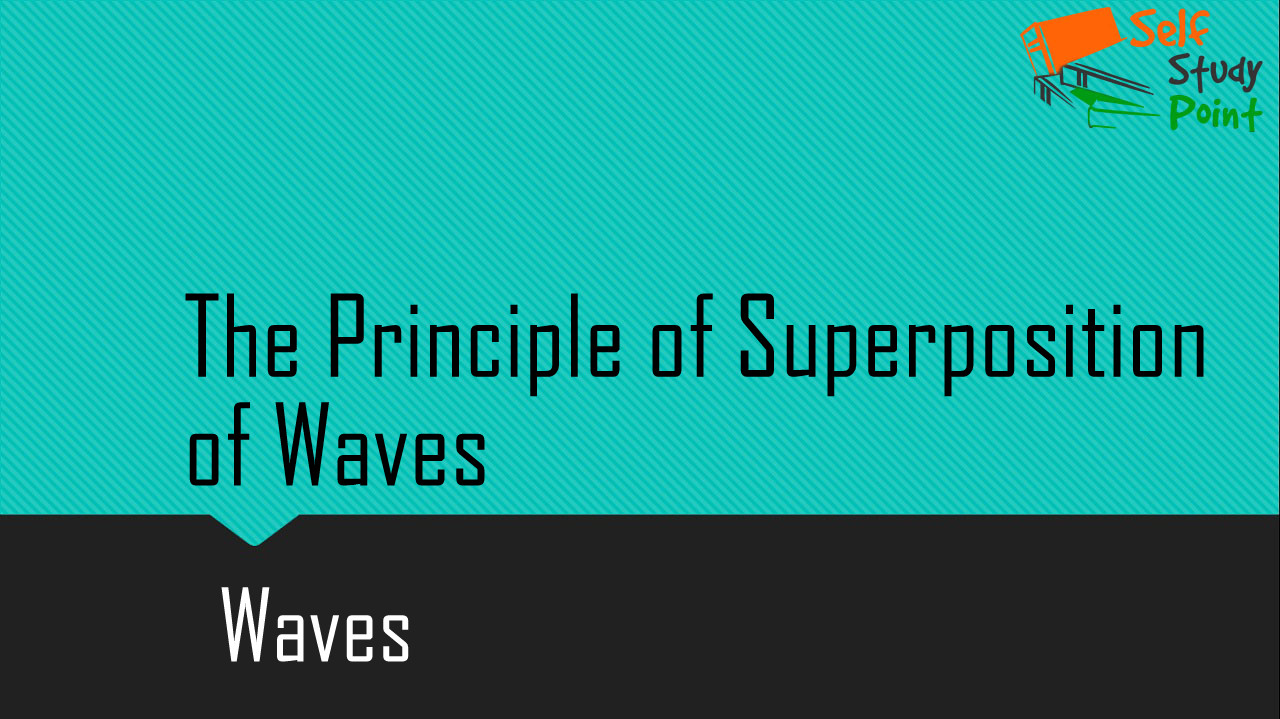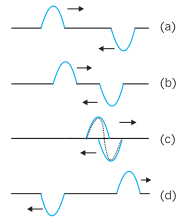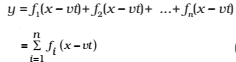## The Principle of Superposition of Waves

The net displacement of any element of the string at a given time is the algebraic sum of the displacements due to each wave. This way of addition of individual waveforms to determine the net waveform is called the principle of superposition.A sequence of pictures depicting two pulses traveling in opposite directions along a stretched string. They meet and pass through each other and move on independently as shown by the sequence of time snapshots (a) through (d). The total disturbance is the algebraic sum of the displacements due to each pulse. When the two disturbances overlap they give a complicated pattern as shown in (c). In region (d) they have passed each other and proceed unchanged.

The principle of superposition can also be expressed by stating that overlapping waves algebraically add to produce a resultant wave (or a net wave). The principle implies that the overlapping waves do not, in any way, alter the travel of each other.

Then the wave function describing the disturbance in the medium isThe superposition of two waves may lead to following three different effects:

1. When two waves of the same frequency moving with the same speed in the same direction in a medium superpose on each other, they give rise to an effect called interference of waves.
2. When two waves of same frequency moving with the same speed in the opposite directions in a medium superpose on each other, they produce stationary waves.
3. When two waves of slightly different frequencies moving with the same speed in the same direction in a medium superpose on each other, they produce beats.

Click on a star to rate it!

Average rating / 5. Vote count:

No votes so far! Be the first to rate this post.